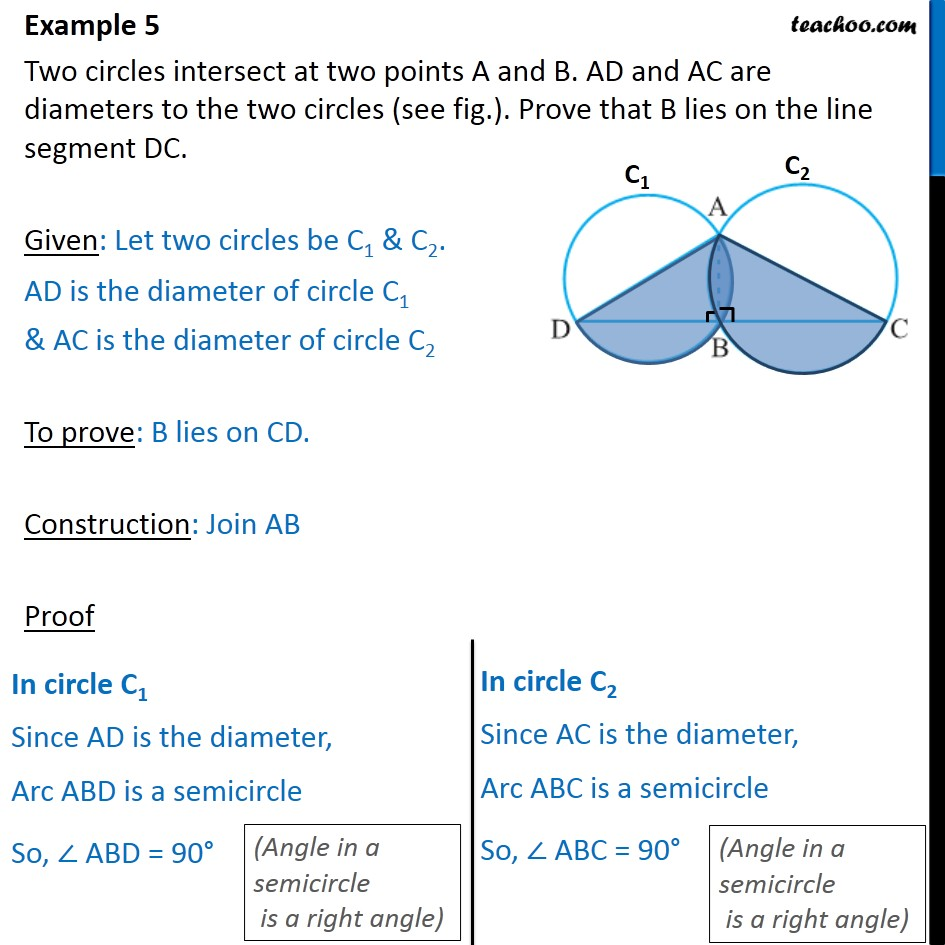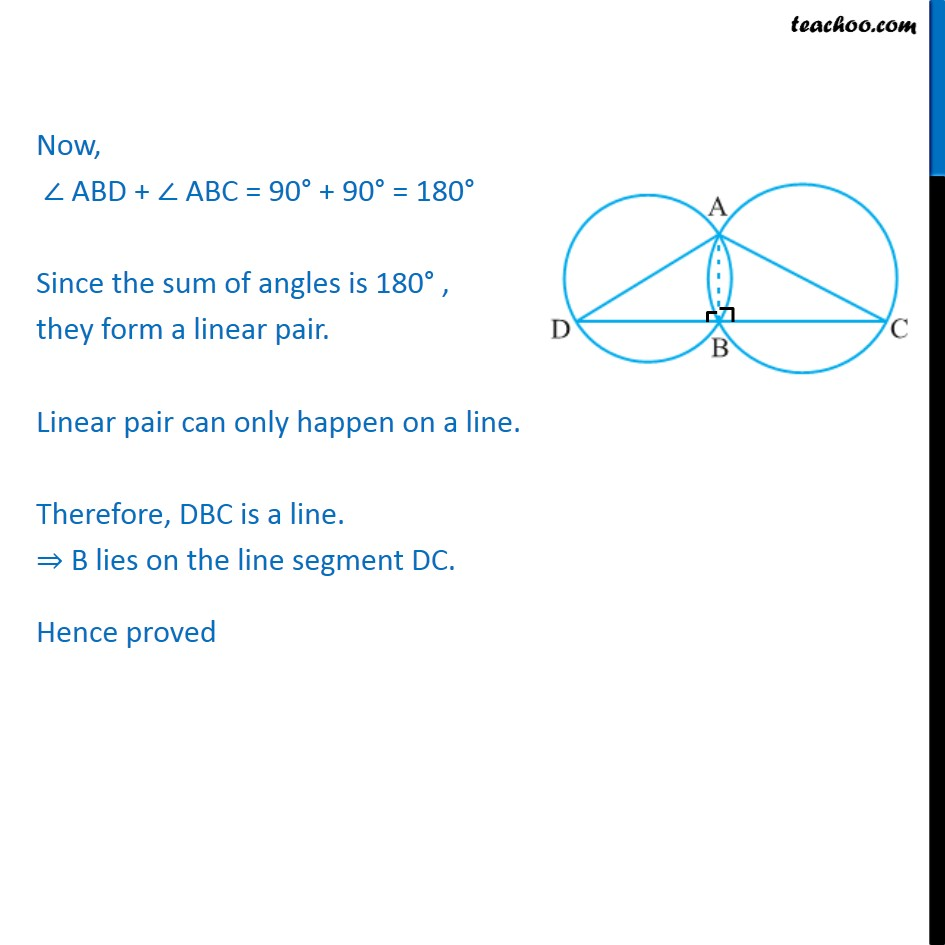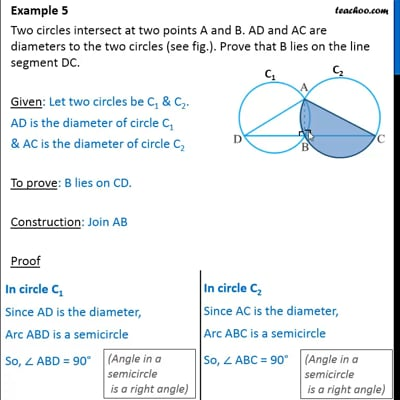Examples

Chapter 10 Class 9 Circles (Term 2)
Serial order wiseThis video is only available for Teachoo black users

### Transcript

Example 5 Two circles intersect at two points A and B. AD and AC are diameters to the two circles (see fig.). Prove that B lies on the line segment DC. Given: Let two circles be C1 & C2. AD is the diameter of circle C1 & AC is the diameter of circle C2 To prove: B lies on CD. Construction: Join AB Proof Now, ABD + ABC = 90 + 90 = 180 Since the sum of angles is 180 , they form a linear pair. Linear pair can only happen on a line. Therefore, DBC is a line. B lies on the line segment DC. Hence proved